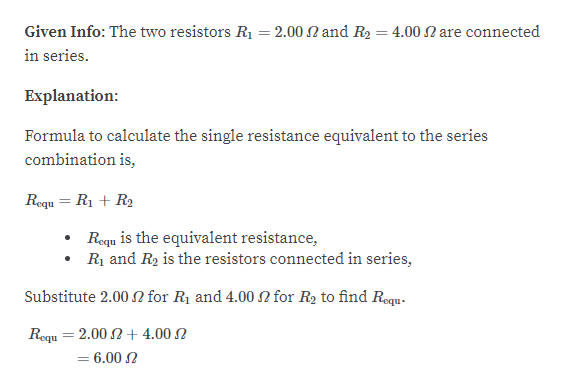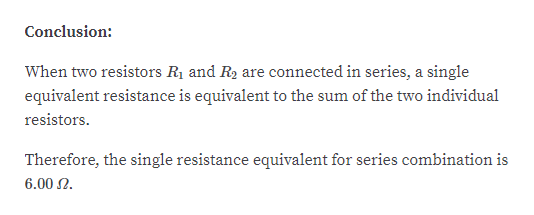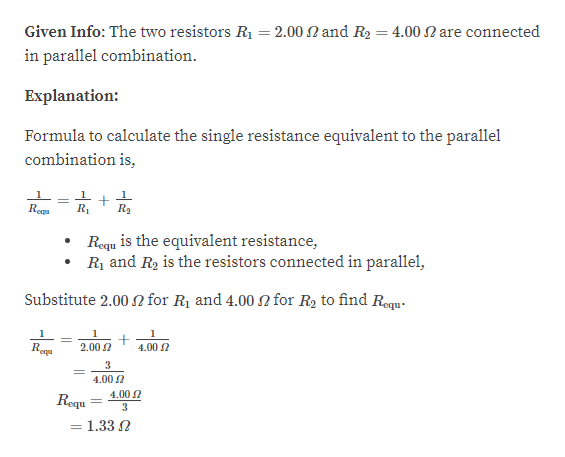# Two resistors, R1 and R2, are connected in series. (a) If R1 =2.00 Ω and R2 = 4.00 Ω, calculate the single resistance equivalentto the series combination. (b) Repeat the calculation fora parallel combination of R1 and R2.

Question
37 views

Two resistors, R1 and R2, are connected in series. (a) If R1 =
2.00 Ω and R2 = 4.00 Ω, calculate the single resistance equivalent
to the series combination. (b) Repeat the calculation for
a parallel combination of R1 and R2.

check_circle

Step 1help_outlineImage TranscriptioncloseGiven Info: The two resistors R = 2.00 N and R2 = 4.00 2 are connected in series. Explanation: Formula to calculate the single resistance equivalent to the series combination is, Requ = R1 + R2 Requ is the equivalent resistance, R and R2 is the resistors connected in series, N for R2 to find Requ- Substitute 2.00 N for R1 and 4.00 Requ = 2.00 2 +4.00 N = 6.00 N fullscreen
Step 2help_outlineImage TranscriptioncloseConclusion: When two resistors R1 and R2 are connected in series, a single equivalent resistance is equivalent to the sum of the two individual resistors. Therefore, the single resistance equivalent for series combination is 6.00 2. fullscreen
Step 3help_outlineImage TranscriptioncloseGiven Info: The two resistors R = 2.00 N and R2 = 4.00 2 are connected in parallel combination. Explanation: Formula to calculate the single resistance equivalent to the parallel combination is, R2 %3D Reg R, Requ is the equivalent resistance, • Rị and R, is the resistors connected in parallel, N for R1 and 4.00 N for R2 to find Requ- Substitute 2.00 Re 4.00 2 2.00 2 4.00 2 4.00 2 Requ = 1.33 2 %3D fullscreen

### Want to see the full answer?

See Solution

#### Want to see this answer and more?

Solutions are written by subject experts who are available 24/7. Questions are typically answered within 1 hour.*

See Solution
*Response times may vary by subject and question.
Tagged in

### Current Electricity